Next: Angular momentum of an Up: Angular momentum Previous: Introduction

## Angular momentum of a point particle

Consider a particle of mass, position vector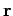, and instantaneous velocity, which rotates about an axis passing through the origin of our coordinate system. We know that the particle's linear momentum is written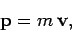(414)

and satisfies(415)

where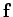is the force acting on the particle. Let us search for the rotational equivalent of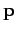.

Consider the quantity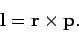(416)

This quantity--which is known as angular momentum--is a vector of magnitude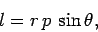(417)

whereis the angle subtended between the directions ofand. The direction of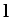is defined to be mutually perpendicular to the directions ofand, in the sense given by the right-hand grip rule. In other words, if vectorrotates onto vector(through an angle less than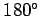), and the fingers of the right-hand are aligned with this rotation, then the thumb of the right-hand indicates the direction of. See Fig. 85.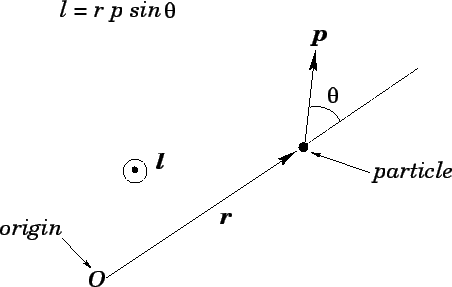Let us differentiate Eq. (416) with respect to time. We obtain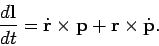(418)

Note that the derivative of a vector product is formed in much the same manner as the derivative of an ordinary product, except that the order of the various terms is preserved. Now, we know that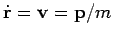and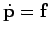. Hence, we obtain(419)

However,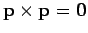, since the vector product of two parallel vectors is zero. Also,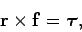(420)

where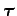is the torque acting on the particle about an axis passing through the origin. We conclude that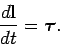(421)

Of course, this equation is analogous to Eq. (415), which suggests that angular momentum,, plays the role of linear momentum,, in rotational dynamics.

For the special case of a particle of massexecuting a circular orbit of radius, with instantaneous velocityand instantaneous angular velocity, the magnitude of the particle's angular momentum is simply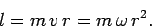(422)Next: Angular momentum of an Up: Angular momentum Previous: Introduction
Richard Fitzpatrick 2006-02-02